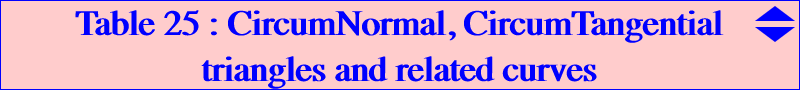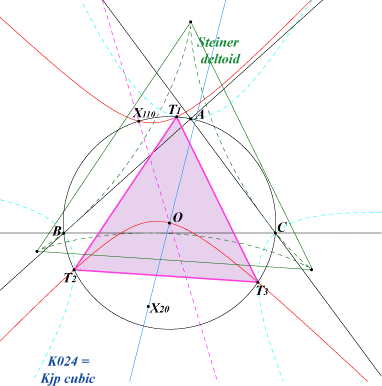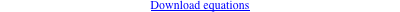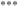These two triangles are very frequently mentioned specially in pages devoted to equilateral cubics. Their vertices lie on many interesting curves as seen below. First we recall some of their properties as in TCCT p.166.CircumTangential Triangle This triangle T1T2T3 is formed by the points on the circumcircle such that the line passing through one point and its isogonal conjugate is tangent to the circumcircle. It is equilateral and homothetic to the Morley triangle. The homothety h(X20, 3/2) maps its vertices to the cusps of the Steiner deltoid. T1, T2, T3 lie on the Kjp cubic and other curves as seen below. The tangential triangle of the CircumTangential triangle is called Stammler triangle, also equilateral.CircumNormal Triangle This triangle N1N2N3 is formed by the points on the circumcircle such that the line passing through one point and its isogonal conjugate is normal to the circumcircle i.e. passes through O. It is equilateral and homothetic to the Morley triangle. The homothety h(X631, -3/2) maps its vertices to the cusps of the Steiner deltoid. N1, N2, N3 lie on the McCay cubic and other curves as seen below. *** These CircumTangential and CircumNormal triangles are symmetric in O. See also the related FG paper "A Morley configuration".Related Conics Any conic passing through O and the vertices of one of these two triangles is obviously a rectangular hyperbola. This gives two pencils of rectangular hyperbolas we call CircumTangential and CircumNormal rectangular hyperbolas denoted by RHT and RHN. Each conic of each pencil is entirely characterized by a fifth point P = u:v:w on the curve. Their equations are rather complicated and can be downloaded here :The following tables give a selection (some of them highlighted) of such hyperbolas with given P on the circumcircle.CircumTangential rectangular hyperbolas RHT CircumNormal rectangular hyperbolas RHNP centers on the curve X(74) X(3), X(74), X(2574), X(2575) X(98) X(2), X(3), X(98), X(3413), X(3414), see below X(102) X(3), X(40), X(102) X(103) X(3), X(103), X(165) X(104) X(3), X(21), X(104), X(3307), X(3308) X(105) X(3), X(55), X(105) X(106) X(1), X(3), X(106) X(111) X(3), X(111), X(574), X(1995) X(477) X(3), X(30), X(477), X(523) X(699) X(3), X(32), X(699) X(713) X(3), X(713), X(1333) X(727) X(3), X(58), X(727) X(729) X(3), X(6), X(729) X(733) X(3), X(39), X(733) X(741) X(3), X(171), X(741) X(759) X(3), X(35), X(759) X(840) X(3), X(840), X(1155) X(842) X(3), X(23), X(842) X(915) X(3), X(28), X(915) X(917) X(3), X(27), X(917) X(933) X(3), X(933), X(1624) X(934) X(3), X(934), X(2283) X(953) X(3), X(513), X(517), X(859), X(953) X(972) X(3), X(972), X(1817) X(1141) X(3), X(5), X(1141) X(1289) X(3), X(1289), X(2409) X(1294) X(3), X(20), X(1294) X(1297) X(3), X(22), X(1297) X(1298) X(3), X(418), X(1298), X(2979) X(1299) X(3), X(24), X(1299) X(1300) X(3), X(4), X(1300) X(1477) X(3), X(57), X(1477) X(2371) X(3), X(9), X(2371) X(2687) X(3), X(1325), X(2687) X(2693) X(3), X(2071), X(2693) X(2697) X(3), X(858), X(2697) X(2698) X(3), X(237), X(511), X(512), X(2698) X(2716) X(3), X(2077), X(2716) X(2718) X(3), X(36), X(2718) X(2724) X(3), X(514), X(516), X(2724) X(2734) X(3), X(515), X(522), X(2734) X(2757) X(3), X(8), X(2757)
 P centers on the curve X(99) X(3), X(99), X(376), X(3413), X(3414) X(100) X(3), X(100), X(3307), X(3308) X(107) X(3), X(4), X(107), X(1075) X(108) X(3), X(56), X(108) X(109) X(1), X(3), X(109), X(1745) X(110) X(3), X(54), X(110), X(182), X(1147), X(1385), X(2574), X(2575), X(6759), X(8717), X(8718), X(8723), X(8907), X(9932), X(11935), X(12584), X(12893), X(13293), X(15577), X(19381), X(22962), X(23358), X(38396), X(40913), X(43975), X(45831), X(46616) X(112) X(3), X(32), X(112), X(378) X(476) X(3), X(30), X(476), X(523) X(691) X(3), X(691), X(2080) X(805) X(3), X(511), X(512), X(805) X(901) X(3), X(513), X(517), X(901) X(925) X(3), X(20), X(925) X(927) X(3), X(514), X(516), X(927) X(930) X(3), X(550), X(930) X(934) X(3), X(934), X(999) X(1291) X(3), X(1157), X(1291) X(1293) X(3), X(40), X(1293) X(1301) X(3), X(24), X(1301) X(1302) X(2), X(3), X(1302) X(1304) X(3), X(186), X(1304) X(1309) X(3), X(515), X(522), X(1309) X(2222) X(3), X(36), X(2222) X(2715) X(3), X(1691), X(2715) X(2720) X(3), X(1319), X(2720) X(2731) X(3), X(944), X(2731) X(2743) X(3), X(2077), X(2743) X(2867) X(3), X(525), X(1503), X(2867)RHT and RHN are the CT- and CN-isogonal transforms of a line (L) passing through O. The two hyperbolas have the same points at infinity (those of the isogonal transform with respect to ABC of the line) and are symmetric with respect to O. The common tangent at O is the line (L) through O. The 4th points of RHT and RHN on (O) are the CT- and CN-isogonal conjugates of its point at infinity, obviously symmetric about O.Remark 1 : the most interesting hyperbola is probably the CircumNormal rectangular hyperbola passing through X(110) since it contains 27 ETC centers and the vertices of the Kosnita triangle (see ETC X1658). Its center is X(1511) and its asymptotes are parallel to those of the Jerabek hyperbola. We meet this hyperbola in table 16 : it is the locus of points whose polar conic in the Neuberg-Lemoine pencil is a circle. It is also the polar conic of X(3) in K361. Its inverse in the circumcircle is the strophoid K725. *** Another remarkable conic is the CircumTangential rectangular hyperbola passing through X(98). It contains 49 ETC centers namely X(2), X(3), X(98), X(3413), X(3414), X(6194), X(6222), X(6287), X(6295), X(6308), X(6312), X(6316), X(6399), X(6582), X(8182), X(8295), X(8667), X(8669), X(9751), X(9756), X(9888), X(10810), X(11155), X(13708), X(13828), X(14830), X(22869), X(22914), X(31981), X(33370), X(33371), X(33388), X(33389), X(33420), X(33421), X(37619), X(39647), X(40923), X(42667), X(42668), X(48932), X(49832), X(49833), X(49881), X(49882), X(49923), X(49924), X(49963), X(49964). Its center is the midpoint X(12042) of X(3)-X(98) and it contains the vertices of the 1st Neuberg triangle. Remark 2 : the yellow, light blue, orange cells correspond to hyperbolas homothetic to the Jerabek, Kiepert, Feuerbach hyperbolas respectively. *** Note that the inversive image in the circumcircle of any of the rectangular hyperbolas gives a strophoid passing through the vertices of one of the two triangles. See K725 for example in remark 1 above. The CircumTangential rectangular hyperbolas are the images of lines passing through G under the involution f defined in the page K024.Cubics The following tables give a selection of remarkable cubics through the vertices of these triangles.CircumTangential cubics CircumNormal cubicscubic other centers on the curve, remark K024 none, a nK0 K078 1, 2, 3, 165, 5373, 6194, 21214, 32524 K085 1, 23830, 23831, 23832, 23833, 23834, 23835, 23836, 23837, a cK K098 none, a nK60 K105 3, 4, 15328, 15329, a nK60 K403 none, a psK K409 none, a nK K686 2, 6, 34203, 34204 a nK60 K723 3, 15329, 23832, 41337, 46608 K726 6, 8617 K727 2, 3, 7712, 39162, 39163, 39164, 39165 K728 2, 3, 23, 111, 187, 2930, 3098, 9999, 10546, 11645, 14830 K734 2, 3, 4230, a nK K735 3, 182, 3098, 8666, 8715 K896 6, 111, 368, 511, 3098, 5640, 6194 K1063 1, 3, 8, 40, 21214, 21227, 21228, 21229, 21306, 21307 K1064 3, 15, 16, 98, 385, 21444 K1098 3, 194, 39641, 39642 K1114 2, 3, 6, 3098, 8667, 33705, 33707, 33708 K1161 3, 6, 40122 K1265 2, 3, 55, 574, 4550, 47047, 47048, 47049, 47050, 47051 K1266 2, 3, 154, 3357, 9756, 47052 K1267 3, 3357, 5450, 6759, 6796 K1268 3, 54, 7691 K1269 3, 1147, 7689 K1290 2, 182, 4550, 7709 K1291 3, 32, 3098, 24309 K1320 3, 4240, 14966, 15328, 23981, 43083 K1336 2, 3, 20, 2979, 6194, 57450, 57451
 cubic other centers on the curve, remark K003 McCay cubic, a pK K009 Lemoine cubic, a psK K227 only one vertex on each curve K361 3, 4, 54, 1342, 1343, 14371, 38808, a psK K373 3, 54, 96, 1147, 39641, 39642, a pK K404 none, nK0(X924, X323) K405 3, 4, 64, 1676, 1677, 6759, 42457, McCay-Lemoine cubic, a spK. D(1/2), see Table 33 K519 254, 1147, an Orion cubic. See Table 11 K664 3, 4, 83, 8718, 16835, 41367, isogonal transform of K665, a spK K725 3, 30, 110, 1157, 2080, 6760, 8724, 13557, 14072, 14933, 14934, 22765, 23240, Neuberg strophoid K735 3, 182, 3098, 8666, 8715 K736 3, 40, 376, 3576, 7709 K931 3, 6, 30, 67, 182, 186, 12584, 15364, 15365 K1267 3, 3357, 5450, 6759, 6796 K1268 3, 54, 7691 K1269 3, 1147, 7689 K1270 3, 15, 16, 99, 11676, 21444 K1290 2, 182, 4550, 7709 K1328 3, 5, 8718, 33541

The orange cells contain cubics with the same infinite points as the McCay cubic K003

See K735 for explanations on light-blue cells.pK cubics The loci of poles, pivots and isopivots of CircumNormal pKs are K378, K361 and K405 respectively. The loci of poles, pivots and isopivots of CircumTangential pKs are K402, K403 and K902 respectively. nK0 cubics The CircumTangential nK0s must have their pole either – on the circumconic with perspector X(32) in which case the cubic decomposes into the circumcircle and a line isoconjugate of the circumcircle. The root must lie on the circumcircle. – on the Brocard axis. The root lies on the line GK and the line passing through X(110) and the pole. See K024 for example, also nK0(X511, X323). The CircumNormal nK0s must have their pole either – on the circumconic with perspector X(32) in which case the cubic decomposes into the circumcircle and a line isoconjugate of the circumcircle. The root must lie on the circumcircle. – on the line X(50)X(647). The root lies on the line X(323)X(401). These two lines meet at X(2623), the crossconjugate of X(115) and X(6). K404 = nK0(X924, X323) is an example of such cubic. psK cubics For any pseudo-pole Ω, one can always find one CircumNormal psK and one CircumTangential psK with respective pseudo-pivots : a(X311 x Ω) x Ω ÷ X6 and a(X850 x Ω) x Ω ÷ X6, where x and ÷ are the barycentric product and quotient, aX is the anticomplement of X. Similarly, for any pseudo-pivot P, one can always find one CircumNormal psK and one CircumTangential psK with respective pseudo-poles : a(X5 x P) x P x X6 and a(X523 x P) x P x X6. These psKs become pKs when Ω and P lie on the loci mentioned above. The isogonal transforms of K071 and K670 are two other examples of CircumNormal psKs.Higher degree curves The following tables give a selection of remarkable curves through the vertices of the triangles.CircumTangential curves CircumNormal curves